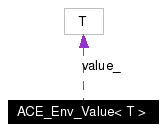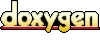# ACE_Env_Value< T > Class Template Reference

Environment Variable Value. More...

`#include <Env_Value_T.h>`

Collaboration diagram for ACE_Env_Value< T >:[legend]
List of all members.

## Public Member Functions

ACE_Env_Value (void)
ACE_Env_Value (const ACE_TCHAR *varname, const T &vardefault)
Constructor that calls .

~ACE_Env_Value (void)
Destroy the value.

operator T (void)
Returns the value as type T.

void open (const ACE_TCHAR *varname, const T &defval)
const ACE_TCHARvarname (void) const
Returns the name of the variable being tracked.

## Private Member Functions

ACE_Env_Value (const ACE_Env_Value< T > &)
Disallow copying and assignment.

ACE_Env_Value< T > operator= (const ACE_Env_Value< T > &)
void fetch_value (void)

## Private Attributes

const ACE_TCHARvarname_
value_

## Detailed Description

### template<class T> class ACE_Env_Value< T >

Environment Variable Value.

Reads a variable from the user environment, providing a default value.

Definition at line 41 of file Env_Value_T.h.

## Constructor & Destructor Documentation

 template ACE_INLINE ACE_Env_Value< T >::ACE_Env_Value ( void )
 Default constructor which isn't bound to a specific environment variable name or a default value. Before being useful it must 'd. Definition at line 12 of file Env_Value_T.inl. ```00013 : varname_ (0) 00014 { 00015 } ```

 template ACE_INLINE ACE_Env_Value< T >::ACE_Env_Value ( const ACE_TCHAR * varname, const T & vardefault )
 Constructor that calls . Definition at line 18 of file Env_Value_T.inl. References ACE_TCHAR, and ACE_Env_Value< T >::fetch_value(). ```00020 : varname_ (varname), 00021 value_(defval) 00022 { 00023 this->fetch_value (); 00024 } ```

 template ACE_INLINE ACE_Env_Value< T >::~ACE_Env_Value ( void )
 Destroy the value. Definition at line 56 of file Env_Value_T.inl. ```00057 { 00058 } ```

 template ACE_Env_Value< T >::ACE_Env_Value ( const ACE_Env_Value< T > & ) ` [private]`
 Disallow copying and assignment.

## Member Function Documentation

 template ACE_INLINE void ACE_Env_Value< T >::fetch_value ( void ) ` [private]`
 Definition at line 36 of file Env_Value_T.inl. Referenced by ACE_Env_Value< T >::ACE_Env_Value(), and ACE_Env_Value< T >::open(). ```00037 { 00038 #if defined (ACE_WIN32) 00039 const ACE_TCHAR *env = ACE_OS::getenv (this->varname_); 00040 if (env != 0) 00041 ACE_Convert (env, value_); 00042 #else 00043 char *nenv = ACE_OS::getenv (ACE_TEXT_ALWAYS_CHAR (this->varname_)); 00044 if (nenv != 0) 00045 ACE_Convert (ACE_TEXT_CHAR_TO_TCHAR (nenv), this->value_); 00046 #endif 00047 } ```

 template ACE_INLINE void ACE_Env_Value< T >::open ( const ACE_TCHAR * varname, const T & defval )
 The constructor, read varname from the environment, using defval as its value if it is not defined. Definition at line 27 of file Env_Value_T.inl. ```00029 { 00030 this->varname_ = varname; 00031 this->value_ = defval; 00032 this->fetch_value (); 00033 } ```

 template ACE_BEGIN_VERSIONED_NAMESPACE_DECL ACE_INLINE ACE_Env_Value< T >::operator T ( void )
 Returns the value as type T. Definition at line 6 of file Env_Value_T.inl. ```00007 { 00008 return value_; 00009 } ```

 template ACE_Env_Value ACE_Env_Value< T >::operator= ( const ACE_Env_Value< T > & ) ` [private]`

 template ACE_INLINE const ACE_TCHAR * ACE_Env_Value< T >::varname ( void ) const
 Returns the name of the variable being tracked. Definition at line 50 of file Env_Value_T.inl. References ACE_Env_Value< T >::varname_. ```00051 { 00052 return this->varname_; 00053 } ```

## Member Data Documentation

 template T ACE_Env_Value< T >::value_` [private]`
 Definition at line 76 of file Env_Value_T.h.

 template const ACE_TCHAR* ACE_Env_Value< T >::varname_` [private]`
 Definition at line 75 of file Env_Value_T.h. Referenced by ACE_Env_Value< T >::open(), and ACE_Env_Value< T >::varname().

The documentation for this class was generated from the following files:
Generated on Thu Nov 9 11:22:01 2006 for ACE by1.3.6